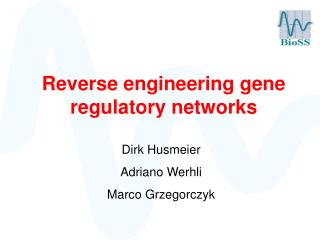DownloadDownload PresentationReverse engineering gene regulatory networks

# Reverse engineering gene regulatory networks

Télécharger la présentation## Reverse engineering gene regulatory networks

- - - - - - - - - - - - - - - - - - - - - - - - - - - E N D - - - - - - - - - - - - - - - - - - - - - - - - - - -
##### Presentation Transcript

1. Reverse engineering gene regulatory networks Dirk Husmeier Adriano Werhli Marco Grzegorczyk

2. Systems biology Learning signalling pathways and regulatory networks from postgenomic data

3. unknown

4. unknown high-throughput experiments postgenomic data

5. unknown data data machine learning statistical methods

6. extracted network true network Does the extracted network provide a good prediction of the true interactions?

7. Reverse Engineering of Regulatory Networks • Can we learn the network structure from postgenomic data themselves? • Statistical methods to distinguish between • Direct interactions • Indirect interactions • Challenge: Distinguish between • Correlations • Causal interactions • Breaking symmetries with active interventions: • Gene knockouts (VIGs, RNAi)

8. direct interaction common regulator indirect interaction co-regulation

9. Relevance networks • Graphical Gaussian models • Bayesian networks

10. Relevance networks • Graphical Gaussian models • Bayesian networks

11. Relevance networks(Butte and Kohane, 2000) • Choose a measure of association A(.,.) • Define a threshold value tA • For all pairs of domain variables (X,Y) compute their association A(X,Y) 4. Connect those variables (X,Y) by an undirected edge whose association A(X,Y) exceeds the predefined threshold value tA

12. Association scores

13. 1 2 ‘direct interaction’ X 1 2 1 2 X X ‘common regulator’ 1 1 2 2 ‘indirect interaction’ strong correlation σ12

14. Pairwise associations without taking the context of the system into consideration

15. Relevance networks • Graphical Gaussian models • Bayesian networks

16. 1 2 direct interaction 1 2 Graphical Gaussian Models strong partial correlation π12 Partial correlation, i.e. correlation conditional on all other domain variables Corr(X1,X2|X3,…,Xn)

17. Distinguish between direct and indirect interactions direct interaction common regulator indirect interaction co-regulation A and B have a low partial correlation

18. 1 2 direct interaction 1 2 Graphical Gaussian Models strong partial correlation π12 Partial correlation, i.e. correlation conditional on all other domain variables Corr(X1,X2|X3,…,Xn) Problem: #observations < #variables

19. Shrinkage estimation and the lemma of Ledoit-Wolf

20. Shrinkage estimation and the lemma of Ledoit-Wolf

21. Graphical Gaussian Models direct interaction common regulator indirect interaction P(A,B)=P(A)·P(B) But: P(A,B|C)≠P(A|C)·P(B|C)

22. Undirected versus directed edges • Relevance networks and Graphical Gaussian models can only extract undirected edges. • Bayesian networks can extract directed edges. • But can we trust in these edge directions? It may be better to learn undirected edges than learning directed edges with false orientations.

23. Relevance networks • Graphical Gaussian models • Bayesian networks

24. Bayesian networks • Marriage between graph theory and probability theory. • Directed acyclic graph (DAG) representing conditional independence relations. • It is possible to score a network in light of the data: P(D|M), D:data, M: network structure. • We can infer how well a particular network explains the observed data. NODES A B C EDGES D E F

25. Bayesian networks versus causal networks Bayesian networks represent conditional (in)dependence relations - not necessarily causal interactions.

26. Node A unknown A A True causal graph B C B C Bayesian networks versus causal networks

27. Bayesian networks versus causal networks A A A B C B C B C • Equivalence classes: networks with the same scores: P(D|M). • Equivalent networks cannot be distinguished in light of the data. A B C

28. A C B Equivalence classes of BNs A C B A C A B P(A,B)≠P(A)·P(B) P(A,B|C)=P(A|C)·P(B|C) C B A C completed partially directed graphs (CPDAGs) B v-structure A P(A,B)=P(A)·P(B) P(A,B|C)≠P(A|C)·P(B|C) C B

29. Symmetry breaking A A A B C B C B C A • Interventions • Priorknowledge B C

30. Symmetry breaking A A A B C B C B C A • Interventions • Priorknowledge B C

31. Interventional data A and B are correlated A B inhibition of A A B A B A B down-regulation of B no effect on B

32. Learning Bayesian networks from data P(M|D) = P(D|M) P(M) / Z M: Network structure. D: Data

33. Learning Bayesian networks from data P(M|D) = P(D|M) P(M) / Z M: Network structure. D: Data

34. Evaluation • On real experimental data, using the gold standard network from the literature • On synthetic data simulated from the gold-standard network

35. Evaluation • On real experimental data, using the gold standard network from the literature • On synthetic data simulated from the gold-standard network

36. From Sachs et al., Science 2005

37. Evaluation: Raf signalling pathway • Cellular signalling network of 11 phosphorylated proteins and phospholipids in human immune systems cell • Deregulation  carcinogenesis • Extensively studied in the literature  gold standard network

38. Raf regulatory network From Sachs et al Science 2005

39. Flow cytometry data • Intracellular multicolour flow cytometry experiments: concentrations of 11 proteins • 5400 cells have been measured under 9 different cellular conditions (cues) • Downsampling to 100 instances (5 separate subsets): indicative of microarray experiments